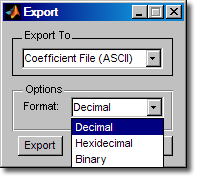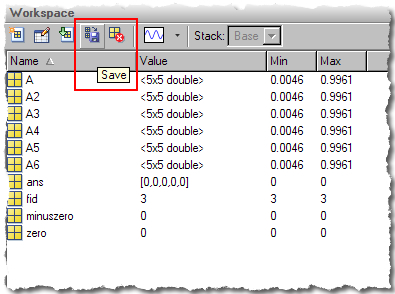How to be Top Mathematician

I consider myself to be a pretty good cook, but I am no Top Chef. On Top Chef the contestants have to cook their ingredients perfectly, which requires single-degree control of the temperature on their stoves and cook times exact down to seconds. For me, I’m competing only for survival and not money so I’ll take delicious and good enough over perfect; if it comes out a little rubbery, so what?

Computers, on the other hand, don’t have a native concept of “good enough.” Computers demand perfection and precision. Fortunately programming languages (such as MATLAB) provide easy ways for us to easily achieve a certain level of precision and repeatably.

It’s not always easy to determine the precision you’re using. For instance to those unfamiliar with IEEE floating point representation, the following might have surprising results:

0.1 + 0.1 + 0.1 - 0.3
ans =

5.5511e-017

Why do we get this result, when we expect 0? At first glance the two numbers look exactly the same:

0.1 + 0.1 + 0.1
0.3
ans =

0.3000

ans =

0.3000

Fortunately the Command Window has a special function to help us understand what is going on. The format function controls how numbers are represented in Command Window output.

At first we might try long format.

format long
0.1 + 0.1 + 0.1
0.3
ans =

0.300000000000000

ans =

0.300000000000000

The long format gives us lots of extra digits, but that still does not show us the difference between these two numbers. To see the difference, we need to look at the actual 64-bit floating point representation.

format hex
0.1 + 0.1 + 0.1
0.3
ans =

3fd3333333333334

ans =

3fd3333333333333

You can see that these two numbers are close but not exactly the same. The reason for this is to represent numbers on the computer, we have to sample an infinite number of numbers between 10^-308 to 10^+308, leading to round-off. This round-off error is measured by the difference between two consecutive numbers, using the magical function eps. There is a great explanation of this in technical note 1108. This subtle difference between a typed and calculated number can be quite important in program flow, such as with if, for, and while statements. Number representation is also quite important for file I/O.

The most basic text file operations are done using fprintf. We can see that using the default floating point representation we do not get back the same exact numbers that we started with. If these numbers were coefficients, we could destabilize a system before we even begin analysis.

format short
A = rand(5);
fid = fopen('data.dat','w');
fprintf(fid,'%f ',A);
fclose(fid);
fid = fopen('data.dat','r');
A2 = fscanf(fid,'%f ',[5 5]);
fclose(fid);
max(A-A2)
ans =

1.0e-006 *

0.4303    0.4098    0.2859    0.2926    0.4377

Once again, the day is saved with hex notation. There’s a little trickiness here due to my choice of reading in characters through fscanf. If you run this bit at home, compare the two text files. The first one is nice and human readable, but the second one has the best precision for a computer.

fid = fopen('data2.dat','w');
fprintf(fid,'%bx ',A);
fclose(fid);
fid = fopen('data2.dat','r');
A3 = fscanf(fid,'%c ',[16 inf]);
fclose(fid);
A3 = hex2num(A3');
A3 = reshape(A3,5,5);
max(A-A3)
ans =

0     0     0     0     0

This issue can happen with other text file formats as well, such as CSV. If you’re on windows, Microsoft Excel provides a good balance because the files are easily readable, but the data is stored in binary.

csvwrite('data.csv',A);
max(A-A4)

xlswrite('data.xls',A)
max(A-A5)
ans =

1.0e-005 *

0.4092    0.3846    0.2146    0.4540    0.4040

ans =

0     0     0     0     0

Precision considerations are not just for the command line functions. They should be kept in mind when importing/exporting from any of our GUIs and from Simulink models. For example, take one of my favorite toolboxes, the Filter Design Toolbox. Back when I worked in tech support, I helped a customer through this very issue. After exporting his coefficients and importing them into a different tool, he saw a wildly different filter. Below I’ve captured the export window, where there are several different options for output format. Most of our toolbox GUIs have similar options, and in cases where there is not, there is usually a command line function to set the desired precision.If you are only going to and from MATLAB, for best results when saving data, use a MAT-file with the save and load commands. For this you can use the export button in the Workspace Browser.In keeping with the previous series, save and load also have a nice ASCII option:

save data.mat -ascii -double A
max(A-A6)
ans =

0     0     0     0     0

A final fun floating point subtlety; there are two different but equivalent zeroes.

format hex
zero = 0
minuszero = -0
zero =

0000000000000000

minuszero =

8000000000000000

|

コメント

コメントを残すには、ここ をクリックして MathWorks アカウントにサインインするか新しい MathWorks アカウントを作成します。